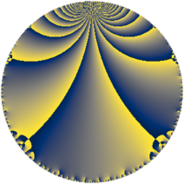# Properties

 Label 50.4.eLevel $50$ Weight $4$ Character orbit 50.e Rep. character $\chi_{50}(9,\cdot)$ Character field $\Q(\zeta_{10})$ Dimension $32$ Newform subspaces $1$ Sturm bound $30$ Trace bound $0$

# Related objects

## Defining parameters

 Level: $$N$$ $$=$$ $$50 = 2 \cdot 5^{2}$$ Weight: $$k$$ $$=$$ $$4$$ Character orbit: $$[\chi]$$ $$=$$ 50.e (of order $$10$$ and degree $$4$$) Character conductor: $$\operatorname{cond}(\chi)$$ $$=$$ $$25$$ Character field: $$\Q(\zeta_{10})$$ Newform subspaces: $$1$$ Sturm bound: $$30$$ Trace bound: $$0$$

## Dimensions

The following table gives the dimensions of various subspaces of $$M_{4}(50, [\chi])$$.

Total New Old
Modular forms 96 32 64
Cusp forms 80 32 48
Eisenstein series 16 0 16

## Trace form

 $$32 q + 32 q^{4} - 30 q^{5} - 12 q^{6} + 26 q^{9} + O(q^{10})$$ $$32 q + 32 q^{4} - 30 q^{5} - 12 q^{6} + 26 q^{9} - 40 q^{10} - 106 q^{11} + 80 q^{12} + 56 q^{14} + 260 q^{15} - 128 q^{16} + 320 q^{17} + 110 q^{19} - 160 q^{20} - 36 q^{21} - 360 q^{22} - 370 q^{23} - 192 q^{24} - 1050 q^{25} + 808 q^{26} - 1200 q^{27} - 120 q^{28} - 10 q^{29} + 160 q^{30} - 486 q^{31} + 2560 q^{33} + 616 q^{34} + 340 q^{35} - 104 q^{36} + 680 q^{37} + 1012 q^{39} + 160 q^{40} - 96 q^{41} - 1020 q^{42} - 136 q^{44} - 1500 q^{45} - 832 q^{46} + 1040 q^{47} + 320 q^{48} - 2076 q^{49} + 400 q^{50} + 884 q^{51} - 2550 q^{53} - 120 q^{54} + 720 q^{55} - 224 q^{56} + 2250 q^{59} + 360 q^{60} + 934 q^{61} + 4200 q^{62} + 4660 q^{63} + 512 q^{64} + 1670 q^{65} + 16 q^{66} - 3780 q^{67} - 628 q^{69} - 2440 q^{70} - 2616 q^{71} - 600 q^{73} - 2584 q^{74} - 4500 q^{75} + 800 q^{76} - 4320 q^{77} - 6640 q^{78} - 2800 q^{79} + 160 q^{80} - 5268 q^{81} + 4050 q^{83} + 624 q^{84} - 1420 q^{85} - 692 q^{86} + 9390 q^{87} - 1680 q^{88} + 4520 q^{89} + 9220 q^{90} + 3764 q^{91} + 1280 q^{92} + 656 q^{94} - 4860 q^{95} - 192 q^{96} + 1710 q^{97} + 3280 q^{98} - 2108 q^{99} + O(q^{100})$$

## Decomposition of $$S_{4}^{\mathrm{new}}(50, [\chi])$$ into newform subspaces

Label Dim $A$ Field CM Traces $q$-expansion
$a_{2}$ $a_{3}$ $a_{5}$ $a_{7}$
50.4.e.a $32$ $2.950$ None $$0$$ $$0$$ $$-30$$ $$0$$

## Decomposition of $$S_{4}^{\mathrm{old}}(50, [\chi])$$ into lower level spaces

$$S_{4}^{\mathrm{old}}(50, [\chi]) \cong$$ $$S_{4}^{\mathrm{new}}(25, [\chi])$$$$^{\oplus 2}$$